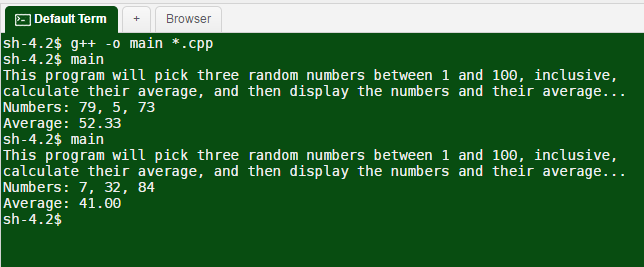# Answered! Write a program named, average.cpp. This program will first print a description of what the program does….

Write a program named, average.cpp. This program will first print a description of what the program does. Actually, ALL programs SHOULD do this as their first step, whether instructions to do so appear in the requirements or not! Next, the program should generate three integers between 1 and 100, inclusive. You will want to verify that you are in fact generating values in this exact
range. Next, calculate the average of these three numbers, taking care to calculate and store the average with accuracy to two decimal places. Finally, display the three randomly generated integers on one line, preceded by a label, and on the following line a label and the value of the average, displayed with exactly two accurate decimal places. Your program should work properly for any three random values in the required range, but here are two examples of what your program could display. Make sure your program’s output is very similar, although your numeric values will be different most of the time.

Example 1:

Don't use plagiarized sources. Get Your Custom Essay on
Answered! Write a program named, average.cpp. This program will first print a description of what the program does….
GET AN ESSAY WRITTEN FOR YOU FROM AS LOW AS \$13/PAGE
```This program will pick three random numbers between 1 and 100, inclusive,
calculate their average, and then display the numbers and their average. . .
Numbers: 36, 82, 16
Average:  44.67
```

Example 2:

```This program will pick three random numbers between 1 and 100, inclusive,
calculate their average, and then display the numbers and their average. . .
Numbers: 79, 94, 31
Average:  68.00
```

Notes:
Make sure you generate numbers in the proper range. Test your program to see that you
generate different numbers each time. This is accomplished by seeding the
generator each time the program runs.
The textbook has an example of doing this. Make sure you output is formatted like
the examples given above.

#include <iostream>

#include <stdlib.h> /* srand, rand */
#include <time.h> /* time */
#include <iomanip> /* set precision */

using namespace std;

//defining main method
int main()
{
cout << “This program will pick three random numbers between 1 and 100, inclusive,” << endl;
cout << “calculate their average, and then display the numbers and their average…” << endl;

srand(time(NULL));
int a, b, c;

a = rand() % 100 + 1;
b = rand() % 100 + 1;
c = rand() % 100 + 1;

float average = ((float)a+b+c)/(3.0);

cout << “Numbers: ” << a << “, ” << b << “, ” << c << endl;
cout << “Average: ” << fixed << setprecision(2) << average << endl;
}